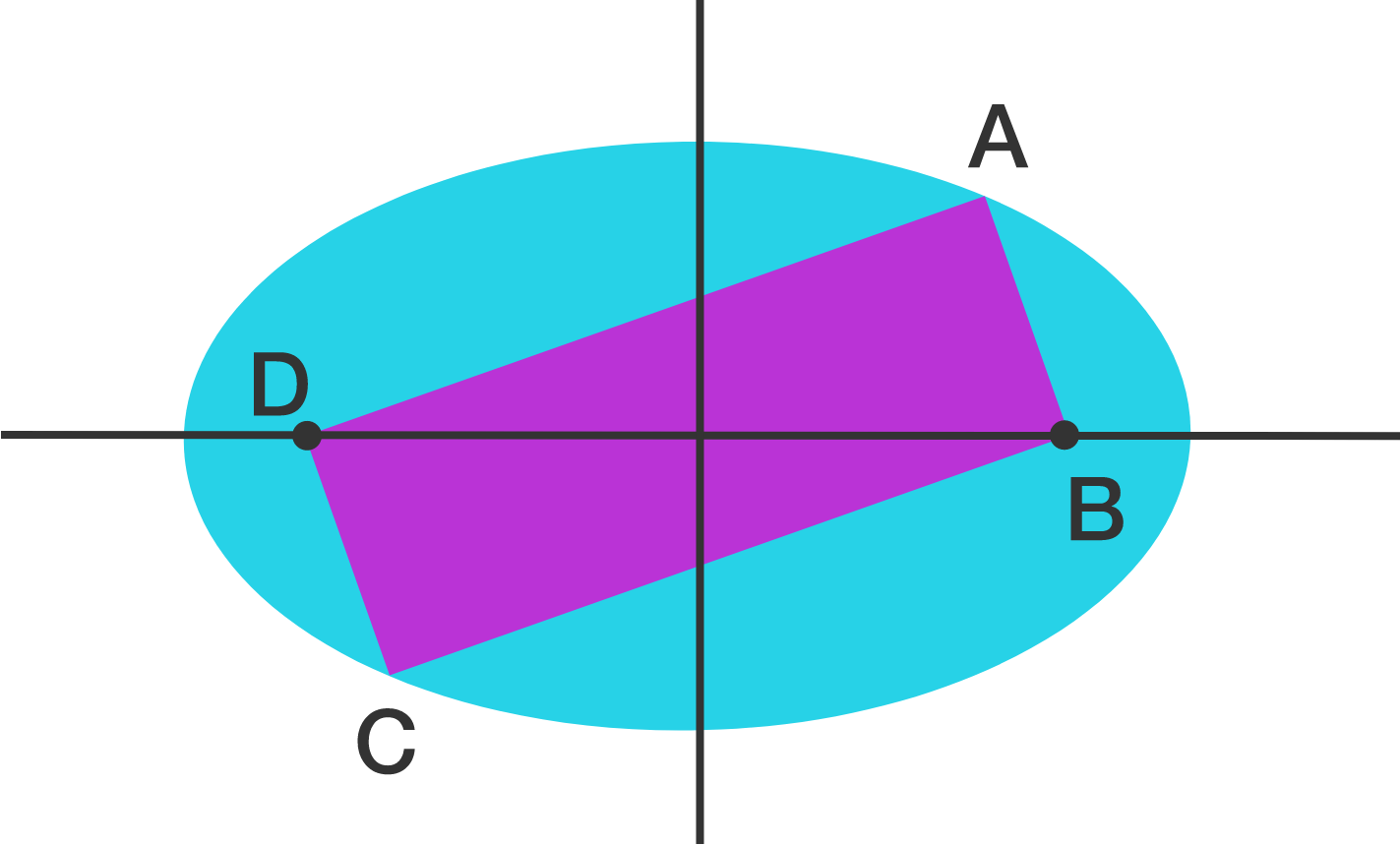# Rectangle in an Ellipse

Geometry Level 2$A,B,C,D$ are consecutive vertices of a rectangle whose area is $2006$ square units. An ellipse with area $2006\pi$, which passes through $A$ and $C$ has its foci at $B$ and $D$.

If the perimeter of the rectangle can be expressed as $p\sqrt{q}$ where $p$ is a positive integer and $q$ is a square-free positive integer, find $p+q$.

×